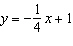Name:    Chapter 3 Practice MC Test

1.

In the figure,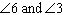are __________.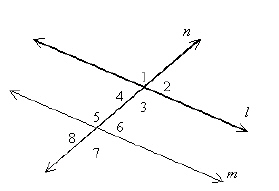a. alternate exterior angles c. corresponding angles b. consecutive interior angles d. alternate interior angles

2.

In the figure,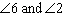are _____________.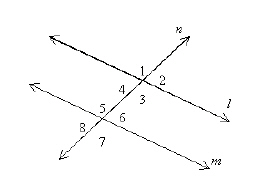a. alternate interior angles c. alternate exterior angles b. consecutive interior angles d. corresponding angles

3.

Find m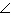1 in the figure below.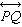and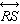are parallel.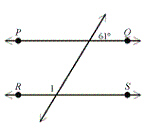a.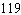° c.° b.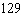° d.°

4.

Use the figure to find the measure of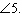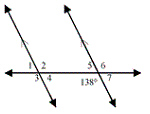a.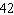° c.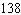° b.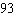° d.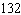°

5.

In the figure below, if l and k are parallel lines, what is the value of x + y?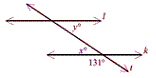a.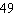° c.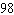° b.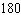° d.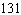°

6.

Line m is parallel to line n and they are each intersected by the same two transversals. Which angle is NOT necessarily congruent to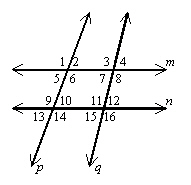a.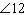c.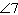b.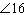d.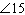7.

Find the value of x that will allow you to prove that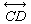below is parallel to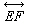if the measure of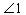is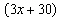° and the measure of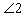is 81°. (The figure may not be drawn to scale.)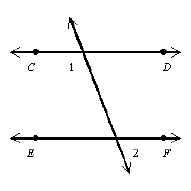a. 22 c. 23 b. 69 d. 36

8.

Which best describes the relationship between the line that passes through (–1, –8) and (4, –4) and the line that passes through (2, –9) and (7, –5)?
 a. parallel b. perpendicular c. same line d. neither perpendicular nor parallel

9.

Which line is parallel to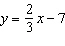?
 a.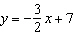c.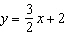b.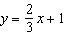d.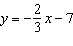10.

Which line is perpendicular to?
 a.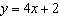c.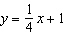b.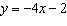d.